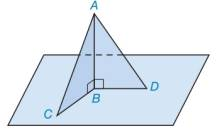Chapter 3.1, Problem 29E### Elementary Geometry for College St...

6th Edition
Daniel C. Alexander + 1 other
ISBN: 9781285195698

#### Solutions

Chapter
Section### Elementary Geometry for College St...

6th Edition
Daniel C. Alexander + 1 other
ISBN: 9781285195698
Textbook Problem
10 views

# In Exercises 27 to 32, use SSS, SAS, ASA, or AAS to prove that the triangles are congruent. Given: A B ¯ ⊥ B C ¯ and A B ¯ ⊥ B D ¯ B C ¯ ≅ B D ¯ Prove: Δ A B C ≅ Δ A B DTo determine

To prove:

The triangle ΔABC is congruent to the triangle ΔABD.

Explanation

Given:

The triangles ΔABC and ΔABD are shown in the figure below,

Figure (1)

From figure (1),

AB¯BC¯ and AB¯BD¯

BC¯BD¯

Property used:

The angle formed by two perpendicular lines is of measure 90°.

Approach:

Consider the triangle,

ΔABC and ΔABD

From the given data,

BC¯BD¯

And the line AB¯ is perpendicular to the lines BC¯ and BD¯ forming right angle for both triangles ΔABC and ΔABD. Thus, ABC and ABD are right angles

### Still sussing out bartleby?

Check out a sample textbook solution.

See a sample solution

#### The Solution to Your Study Problems

Bartleby provides explanations to thousands of textbook problems written by our experts, many with advanced degrees!

Get Started